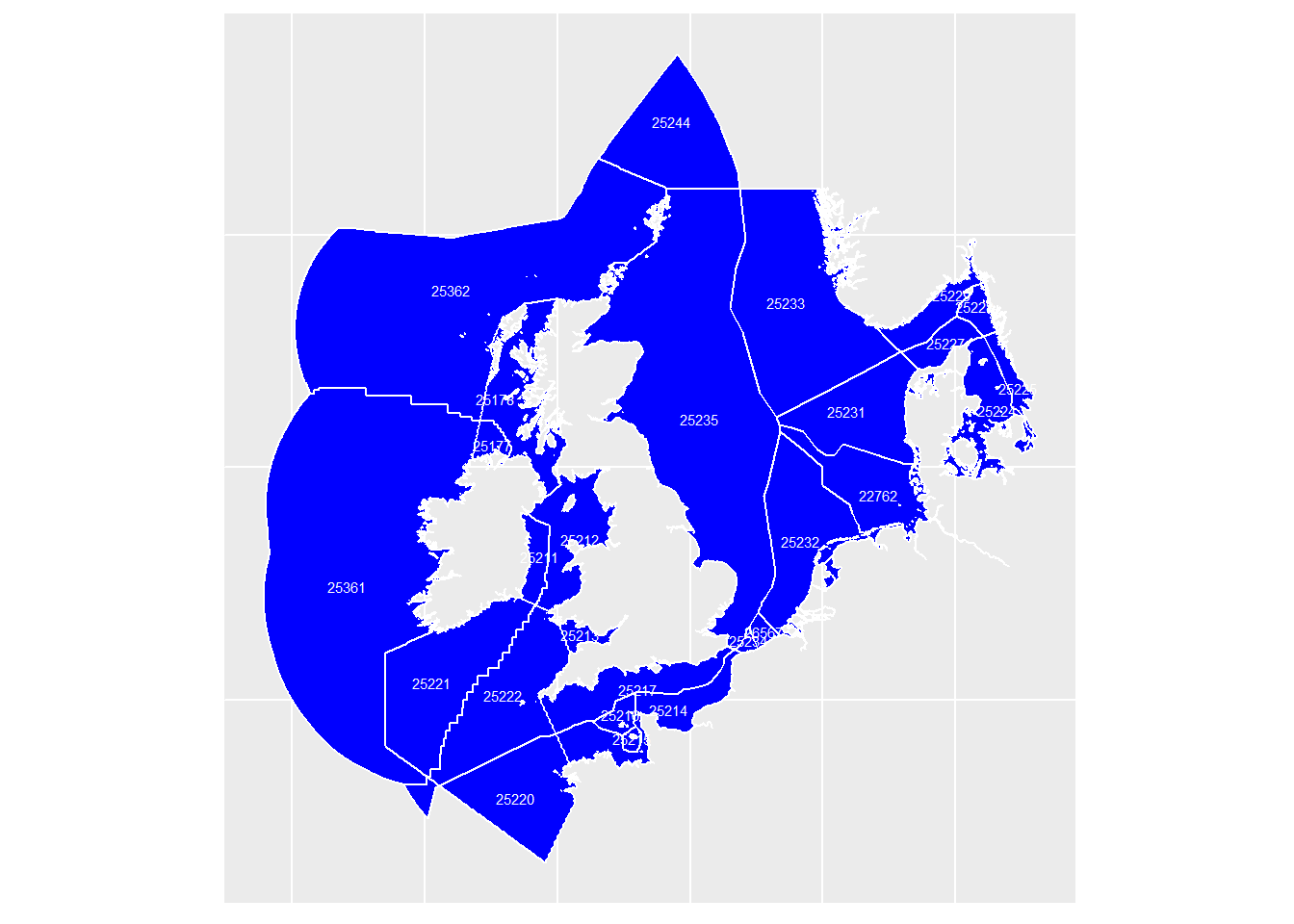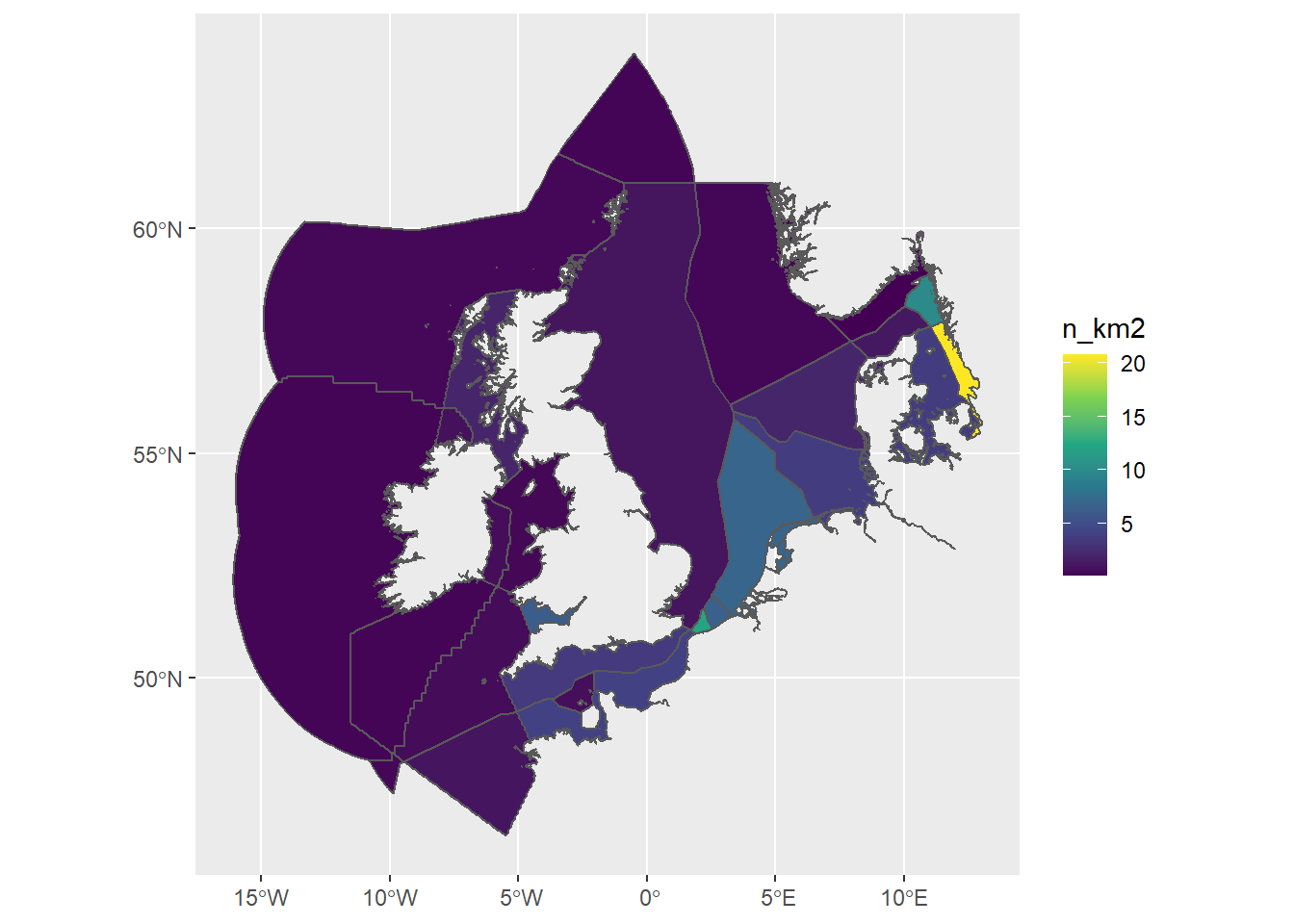``````EMODnetMapCleanStyle <- theme_bw() +
theme(axis.text = element_blank(),
axis.title = element_blank())

EMODnetMapNormalStyle <-
theme(axis.text = element_text(size = 12, color = "blue"),
axis.title = element_text(size = 14, color = "blue"),
legend.text = element_text(size = 10),
legend.position = "right",
legend.key.width = unit(10,"mm"),
legend.key.height = unit(10, "mm"),
plot.background = element_rect(fill = "grey90", colour = "white", size = 0),
panel.border = element_blank()
)``````

# Introduction

## Documentation

Github project: https://github.com/wstolte/mixoplanktonFractions

# Data extraction

## Temporal scale

Data from 1995 until now are considered for the current product.

## Geographical scale

The regions that were selected were assembled from the intersection of the IHO regions and the EEZ from the different countries. These subregions have ID’s that can be used in the WFS query to the EMODnet Biology database.

``````regions <- sf::st_read(quiet = T, dsn = "../data/derived_data/greater_north_sea-selection_from_eez-iho_v4.geojson")
regions %>% ggplot() +
geom_sf(fill = "blue", color = "white") +
geom_sf_text(aes(label = mrgid), size = 2, color = "white") +
theme(axis.title = element_blank(),
axis.text = element_blank(),
axis.ticks = element_blank())``````## Strategy

For each of these subregions, data extraction was done in a two-step approach.

1. Extract phytoplankton data by selecting only observations linkted to the trait “phytoplankton”.
2. The unique datasets obtained in the first steps were inspected, and suitable datasets, which were expected to contain phytoplankton, were selected. The suitable datasets were then downloaded completely (without selection for traits)

## Extraction of complete relevant datasets

Data were extracted from EMODnet Biology via WFS, using the requestData.R script. These data are stored locally and taken into this script here.

``````# all2Data <- read_delim(file.path(dataDir, "all2Data.csv"), delim = ";")

In total, this resulted in 2024331 observations

Per subregion, the number of observations/km2 looks like this:

``````regionN <- all2Data %>%
group_by(mrgid) %>% summarize(n = n()) %>% ungroup() %>%
mutate(mrgid = as.numeric(mrgid))

regions %>% right_join(regionN, by = c(mrgid = "mrgid")) %>%
mutate(n_km2 = n/area_km2) %>%
ggplot() +
geom_sf(aes(fill = n_km2)) +
scale_fill_viridis_c()``````## Join with trophic mode table

Schneider et al. (submitted to Journal of Biological Data, data submitted to WoRMS) has defined trophic mode for about 1500 species of plankton. A first subdivision is in

• phytoplankton - phototrophic, no phagotrophy known
• mixoplankton - capable of phototrophy and phagotrophy
• zooplankton - phagotrophic, no phototrophy known.

Mixoplankton is subdivided in different classes, but at this moment, we will not use this.

``````# read information available in WoRMS traits.
# This is a local copy

trophy <- read_delim("../data/raw_data/traits.csv", delim = ";", guess_max = 10000)``````
``````FALSE
FALSE -- Column specification --------------------------------------------------------
FALSE cols(
FALSE   ScientificName = col_character(),
FALSE   AphiaID = col_character(),
FALSE   Trophy = col_character(),
FALSE   typeMX = col_character(),
FALSE   source = col_character(),
FALSE   sourceType = col_character(),
FALSE   contributor = col_character()
FALSE )``````
``````trophyData <- all2Data %>%
mutate(AphiaID = sub("http://marinespecies.org/aphia.php[?]p=taxdetails&id=", "", aphiaid, )) %>%
full_join(trophy, by = c(AphiaID = "AphiaID")) %>%
select(
mrgid, datasetID, datecollected, decimallongitude, decimallatitude,
scientificname, AphiaID, Trophy, typeMX
) %>%
drop_na(Trophy) %>%
mutate(
season = case_when(
month(datecollected) %in% c(6,7,8) ~ "summer",
month(datecollected) %in% c(3,4,5) ~ "spring",
month(datecollected) %in% c(9,10,11) ~ "autumn",
month(datecollected) %in% c(12, 1, 2) ~ "winter"
)
) %>% drop_na(season) %>%
mutate(season = factor(season, levels = c("winter", "spring", "summer", "autumn")))``````

# Fraction of mixoplankton (first results)

As a first approach, the fraction of mixoplankton/(phytoplankto + mixoplankton) is plotted on a map:

``````plottrophy <- trophyData %>%
group_by(decimallongitude, decimallatitude, Trophy, season) %>%
summarize(n = n()) %>%
filter(Trophy %in% c("mixotroph", "phototroph")) %>%
mutate(fMix = mixotroph/(mixotroph + phototroph)) %>%
drop_na(fMix)``````
``FALSE `summarise()` has grouped output by 'decimallongitude', 'decimallatitude', 'Trophy'. You can override using the `.groups` argument.``
``````write_delim(plottrophy, file.path(csvDir, "trophydata.csv"), delim = ";")

require(rworldxtra)``````
``FALSE Loading required package: rworldxtra``
``````data(countriesHigh)
world <- st_as_sf(countriesHigh) %>%
filter(REGION == "Europe") %>%
filter(ADMIN %in% c("Denmark", "Germany", "Netherlands", "Belgium", "United Kingdom", "France", "Norway", "Sweden", "Ireland")) %>%
``FALSE although coordinates are longitude/latitude, st_intersection assumes that they are planar``
``````ggplot() +